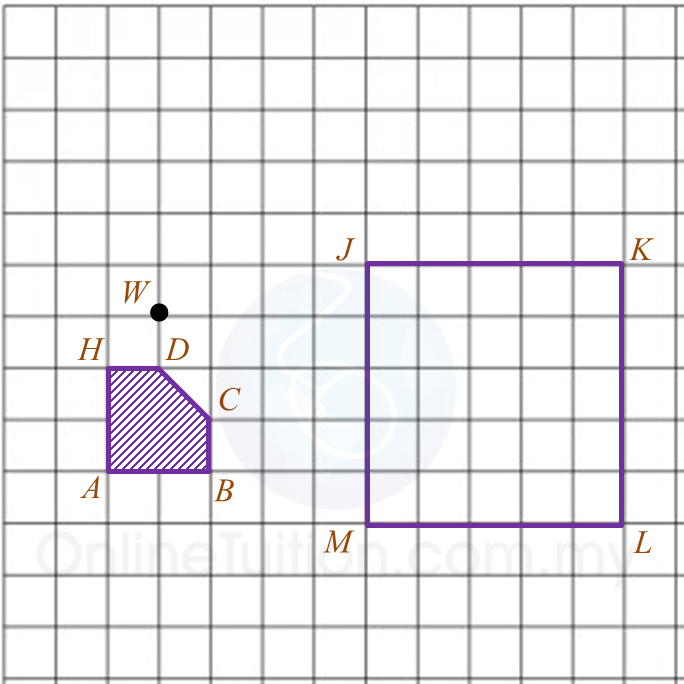# PT3 Mathematics 2016, Question 10

 
Question 10 (a):
Diagram 10.1 is a pie chart showing Ahmad’s monthly expenses.(i) State the mode.
(ii) The following data are the height of students in a class.
132 cm, 125 cm, 137 cm, 130 cm, 128 cm
Calculate the mean.

Solution:
(i) Food

(ii)

 
 
 
Question 10 (b):
Diagram 10.2 shows a polygon HABCD and a square JKLM.
(i) Under a transformation, polygon HABCD will be transformed such that the image will be placed inside the square JKLM. The image formed based on the following conditions:Suggest one possible complete transformation.
(ii) Using the transformation that you have suggested in (b)(i), mark the new position of point W in Diagram 10.2.Solution:
 (i)
 

(ii)Question 10 (c):
Diagram 10.3 shows a car route from P to S. The driver starts the journey from P. When he reaches R, he turns back and travels ¼ the distance of P to R and then he continues to S to finish his journey.Table 10 shows the distance between the destinations.Assuming that the distance travelled in the journey when the driver turns back as a wasted distance, calculate the fraction of the wasted distance to the distance of the whole journey.

Solution:
 

 
 

### 2 thoughts on “PT3 Mathematics 2016, Question 10”

1.Wasted distance is 2x 10KM. since distance is total journey.

•Thanks for pointing out our mistake.